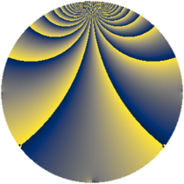# Properties

 Label 81.2.gLevel $81$ Weight $2$ Character orbit 81.g Rep. character $\chi_{81}(4,\cdot)$ Character field $\Q(\zeta_{27})$ Dimension $144$ Newform subspaces $1$ Sturm bound $18$ Trace bound $0$

# Related objects

## Defining parameters

 Level: $$N$$ $$=$$ $$81 = 3^{4}$$ Weight: $$k$$ $$=$$ $$2$$ Character orbit: $$[\chi]$$ $$=$$ 81.g (of order $$27$$ and degree $$18$$) Character conductor: $$\operatorname{cond}(\chi)$$ $$=$$ $$81$$ Character field: $$\Q(\zeta_{27})$$ Newform subspaces: $$1$$ Sturm bound: $$18$$ Trace bound: $$0$$

## Dimensions

The following table gives the dimensions of various subspaces of $$M_{2}(81, [\chi])$$.

Total New Old
Modular forms 180 180 0
Cusp forms 144 144 0
Eisenstein series 36 36 0

## Trace form

 $$144 q - 18 q^{2} - 18 q^{3} - 18 q^{4} - 18 q^{5} - 18 q^{6} - 18 q^{7} - 18 q^{8} - 18 q^{9} + O(q^{10})$$ $$144 q - 18 q^{2} - 18 q^{3} - 18 q^{4} - 18 q^{5} - 18 q^{6} - 18 q^{7} - 18 q^{8} - 18 q^{9} - 18 q^{10} - 18 q^{11} - 18 q^{12} - 18 q^{13} - 18 q^{14} - 18 q^{15} - 18 q^{16} - 18 q^{17} - 9 q^{18} - 18 q^{19} + 18 q^{20} + 9 q^{21} - 18 q^{22} + 9 q^{23} + 36 q^{24} - 18 q^{25} + 45 q^{26} + 9 q^{27} - 9 q^{28} + 9 q^{29} + 36 q^{30} - 18 q^{31} + 36 q^{32} + 9 q^{33} - 18 q^{34} + 9 q^{35} + 18 q^{36} - 18 q^{37} - 9 q^{38} - 18 q^{39} - 18 q^{40} + 27 q^{42} - 18 q^{43} + 54 q^{44} + 36 q^{45} - 18 q^{46} + 36 q^{47} + 81 q^{48} - 18 q^{49} + 99 q^{50} + 45 q^{51} + 45 q^{53} + 108 q^{54} - 9 q^{55} + 126 q^{56} + 36 q^{57} - 18 q^{58} + 45 q^{59} + 99 q^{60} - 18 q^{61} + 81 q^{62} + 36 q^{63} - 18 q^{64} - 18 q^{66} + 9 q^{67} - 99 q^{68} - 72 q^{69} + 36 q^{70} - 90 q^{71} - 234 q^{72} - 18 q^{73} - 162 q^{74} - 108 q^{75} + 63 q^{76} - 162 q^{77} - 135 q^{78} + 36 q^{79} - 288 q^{80} - 90 q^{81} - 36 q^{82} - 90 q^{83} - 243 q^{84} + 36 q^{85} - 162 q^{86} - 162 q^{87} + 63 q^{88} - 81 q^{89} - 99 q^{90} - 18 q^{91} - 144 q^{92} + 36 q^{94} + 18 q^{95} - 27 q^{96} + 9 q^{97} + 81 q^{98} + 54 q^{99} + O(q^{100})$$

## Decomposition of $$S_{2}^{\mathrm{new}}(81, [\chi])$$ into newform subspaces

Label Dim $A$ Field CM Traces $q$-expansion
$a_{2}$ $a_{3}$ $a_{5}$ $a_{7}$
81.2.g.a $144$ $0.647$ None $$-18$$ $$-18$$ $$-18$$ $$-18$$